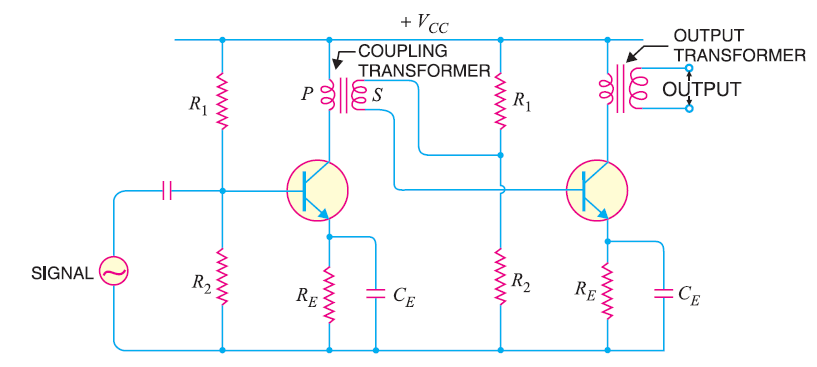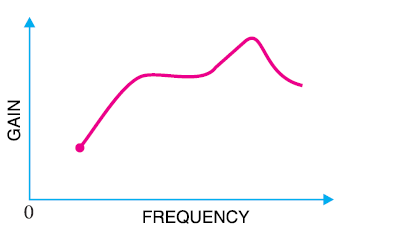# Explain the Working of Transformer Coupled Transistor Amplifier with Circuit Diagram

## Transformer Coupled Transistor Amplifier

As we have already discussed in our previous tutorial, in case of a RC coupled transistor amplifier the voltage and power gain are low since, the effective load resistance of each stage is decreased due to the low resistance presented by the input of each stage to the next stage.

If the effective load resistance of each stage could be increased, the voltage and power gain could also be increased.

This can be achieved by transformer coupling. By using the impedance matching properties of transformer, the low resistance of one stage or load can be reflected as a high load resistance to the previous stage.

Transformer coupling is normally used when the load is small. It is mostly used for power amplification.

### Working of Transformer Coupled Transistor Amplifier

The figure below shows the circuit of a two stage transformer coupled amplifier.As you can see from the above fig. a coupling transformer is used to feed the output of one stage to the input of the next stage.

The primary P of this transformer is made the collector load and its secondary S supplies input to the next stage.

When an a.c. signal is applied to the base of first transistor, it appears in the amplified form across the primary P of the coupling transformer.

Now the voltage developed across P is transferred to the input of the next stage by the transformer secondary S.

The second stage now performs the amplification in an exactly same manner.

### Frequency Response of Transformer Coupled Transistor Amplifier

The frequency response of a transformer coupled amplifier is shown in the figure below.It is clear from the above fig. that its frequency response is poor than the RC coupled amplifier.

The gain is constant only over a small range of frequency.

Since, the output voltage is equal to the collector current multiplied by reactance of primary, hence at low frequencies, as the reactance of primary begins to fall , the output voltage also decrease and hence the gain.

At high frequencies, the capacitance between turns of windings acts as a bypass condenser to reduce the output voltage and hence the gain.

Due to these two factors, there will be disproportionate amplification of frequencies in a complete signal such as music, speech etc.

Hence, transformer coupled amplifier introduces frequency distortion.

### Advantages of Transformer Coupled Transistor Amplifier

1. There is no loss of signal power in the collector or base resistors.
2. An excellent impedance matching can be achieved in a transformer coupled amplifier.
3. Due to excellent impedance matching, transformer coupling provides higher gain. A properly designed single stage transformer coupling can provide the gain of two stages of RC coupling.

### Disadvantages of Transformer Coupled Transistor Amplifier

1. It has a poor frequency response.
2. The coupling transformers are bulky and expensive at audio frequencies.
3. Frequency distortion is higher i.e. low frequency signals are less amplified as compared to the high frequency signals.
4. Transformer coupling introduces hum in the output.

### Application of Transformer Coupled Transistor Amplifier

It is mostly used for impedance matching.

Normally the last stage of a multi stage amplifier is the power stage. Here it is required to transfer maximum power to the output device for example a loud speaker.

Usually the impedance of an output device is few ohms whereas the output impedance of the transistor is several hundred ohms.

In order to match the impedance, a step down transformer of proper turn ratio is used. The impedance of secondary of the transformer is made equal to the load impedance and primary impedance equal to the output impedance of the transistor.

Thus the load on the primary side is comparable to the output impedance of the transistor and this results in maximum power transfer from the transistor to the primary of transformer.

You Might Like The Following Articles